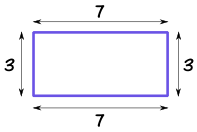Perimeter

Perimeter is the distance around a two-dimensional shape.

Example 1: the perimeter of this rectangle is 7+3+7+3 = 20Example 2: the perimeter of this regular pentagon is 3+3+3+3+3 = 5×3 = 15http://www.mathplayground.com/geoboard.html

http://www.mathlearningcenter.org/web-apps/geoboard/

http://www.funbrain.com/measure/

http://www.sheppardsoftware.com/mathgames/geometry/shapeshoot/PerimeterShapesShoot.htm

http://pbskids.org/cyberchase/math-games/airlines-builder/

http://www.bgfl.org/custom/resources_ftp/client_ftp/ks2/maths/perimeter_and_area/index.html

http://www.mathplayground.com/area_perimeter.html

http://www.factmonster.com/math/knowledgebox/player.html?movie=sfw50955

This entry was posted in Math.